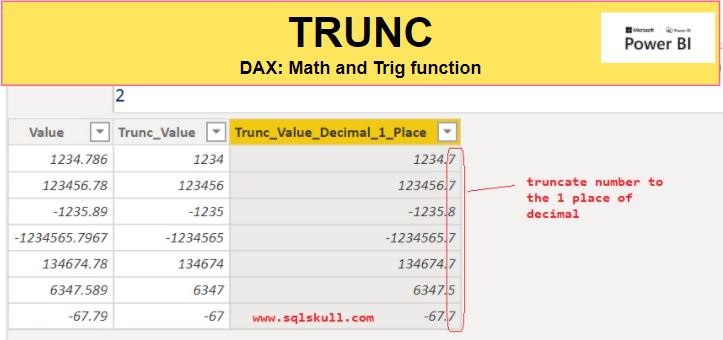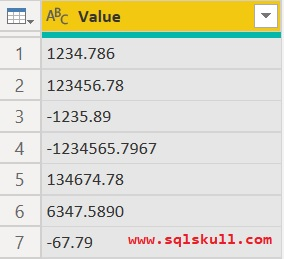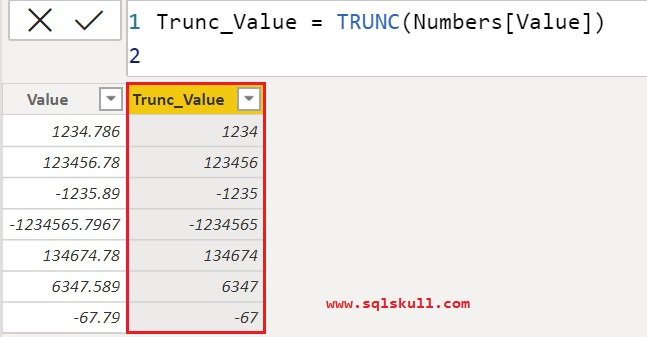Home » TRUNC function DAX

# TRUNC function DAXTRUNC function is a Power BI math and trig function in DAX, which truncates a number to an integer by removing the decimal, or fractional part of the number.

TRUNC function does not round the number down to the nearest integer based on the value of the fractional part of the number.

TRUNC and INT function are similar as both return integers but the only difference is that TRUNC function removes the fractional part of the number while INT rounds numbers down to the nearest integer based on the value of the fractional part of the number.

```SYNTAX
TRUNC(number,num_digits)```
 number is the number you want to truncate. num_digits is a number specifying the precision of the truncation. By default is 0, if not specified

Lets take a look at TRUNC function in Power BI.

Here we have a sample dataset Numbers as shown below.##### Copy sample data :
 Value 1234.786 123456.78 -1235.89 -1234565.7967 134674.78 6347.5890 -67.79

Lets create a calculated column which will remove or truncates a decimal part from numbers in value column and return an integer number.

Trunc_Value = TRUNC(Numbers[Value])You can see, it truncates a number to an integer by removing the decimal part.

#### Specifying the precision of the truncation

You can also specify the precision of the truncation, means you can also truncate the decimal part of number at specified number of places.

Lets truncates the decimal part of number to the 1 place of decimal. For this, you need to specify a second argument num_digits value as 1 to TRUNC function.

Lets create one more calculated column in dataset as shown below.

`Trunc_Value_Decimal_1_Place = TRUNC(Numbers[Value], 1)`You can see, it truncates a number to the 1 place of decimal.

SUM

SUMX

DIVIDEThis site uses Akismet to reduce spam. Learn how your comment data is processed.